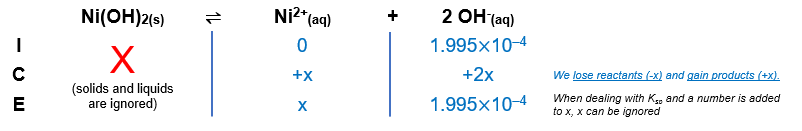# Problem: Calculate the molar solubility of Ni(OH)2 when buffered at pH = 10.3.Express your answer using one significant figure.

###### FREE Expert Solution

Ni(OH)2(s)  Ni2+(aq) + 2 OH(aq)

Calculate the OH concentration in the solution:

$\overline{)\mathbf{pH}\mathbf{+}\mathbf{pOH}\mathbf{=}\mathbf{14}}\phantom{\rule{0ex}{0ex}}\mathbf{10}\mathbf{.}\mathbf{3}\mathbf{+}\mathbf{pOH}\mathbf{=}\mathbf{14}\phantom{\rule{0ex}{0ex}}\mathbf{pOH}\mathbf{=}\mathbf{14}\mathbf{-}\mathbf{10}\mathbf{.}\mathbf{3}$

pOH = 3.7

$\overline{)\mathbf{\left[}{\mathbf{OH}}^{\mathbf{-}}\mathbf{\right]}\mathbf{=}{\mathbf{10}}^{\mathbf{-}\mathbf{pOH}}}\phantom{\rule{0ex}{0ex}}\mathbf{\left[}{\mathbf{OH}}^{\mathbf{-}}\mathbf{\right]}\mathbf{=}{\mathbf{10}}^{\mathbf{-}\mathbf{3}\mathbf{.}\mathbf{7}}$

[OH] = 1.995x10–4 M

Notice that there is a common ion present, OH

We can construct an ICE table for the dissociation of Ni(OH)2The Ksp expression for Ni(OH)2 is:

$\overline{){{\mathbf{K}}}_{{\mathbf{sp}}}{\mathbf{=}}\frac{\mathbf{products}}{\overline{)\mathbf{reactants}}}{\mathbf{=}}\mathbf{\left[}{\mathbf{Ni}}^{\mathbf{2}\mathbf{+}}\mathbf{\right]}{\mathbf{\left[}{\mathbf{OH}}^{\mathbf{-}}\mathbf{\right]}}^{{\mathbf{2}}}}$

We can now plug in the equilibrium concentrations and solve for x, which is the molar solubility of Ni(OH)2 in M or mol/L

80% (83 ratings)###### Problem Details

Calculate the molar solubility of Ni(OH)2 when buffered at pH = 10.3.